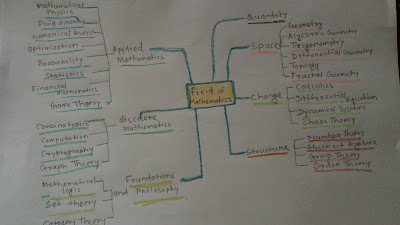# Mathematics and its classification

## Mathematics

Mathematics is all about to have fun. It does not have any specific definition. It may be defined as the science deals with the logic of shape ,quantity and structure.

Mathematics is all around us. It is building block of for everything in our daily lives including mobile device, architecture, art ,money, engineering and even sports. Mathematics is a word which is coming from  Greek language “MATHEMA”. That means knowledge, learning. In simple words Mathematics is a language of understanding. Historically it is known as the science of quality . certain qualities like  power of reasoning,  abstract and spatial thinking, critical thinking, creativity, problem solving ability and even effective communication skills  are nurtured  by  mathematics.

History of Mathematics
Mathematics may also come in some of the primary genres of human knowledge and is as old as human civilization. With the expansion of human life and increasing complexity, mathematics has also expanded and its complexities have also increased. From the simple life of the cave dwellers throughout the history of civilization to the complex and multifaceted humans of modern times, there has been a gradual change in human life. Along with this, mathematics has also developed as a broad and rich branch of human knowledge-science. Although a common man should not have much to deal with the history of mathematics much more than a thousand years behind, but scientists, mathematicians, technologists, economists and many other experts in some form of improved systems of mathematics in everyday life. Use on a vast, unimaginable scale. Nowadays mathematics seems to be ingrained in ubiquitous form with daily life.
How mathematics originated is forgotten in the pages of history today. But we know that 4000 years ago the Babylonian and Egyptian civilizations used mathematics to create an almanac (calendar) so that they had an idea of ​​when the crop should be sown or when the Nile would flood, or She then used it to solve square equations. He was even aware of the theorem which is wrongly credited to Pythagoras. Their cultures were based on agriculture and they needed knowledge of the correct methods for pure drafting and survey of the paths of stars and planets. Arithmetic was used in trade to exchange or keep accounts of money and money. Geometry was used to determine the boundaries around the fields and to build monuments such as pyramids.

Sheath number
Miletus resident Thales (645–546 BCE) is considered the first theoretical mathematician. He said that the height of any object can be measured by comparing it to the shadow cast by the measuring rod. It is believed that he also predicted the occurrence of a solar eclipse. His disciple Pythagoras paved the way for Euclid and Archimedes by making geometry a valid science among Greeks.
The Greeks greatly increased the inherited knowledge of the Babylonian inhabitants. In addition, he also established mathematics as a rational method — a method in which certain basic facts or assumptions are taken to be true (called theorems) to reach conclusions (called subsets or proofs).
It is a matter of pride for India that until the twelfth century, all the significant efforts made for its advancement in the entire development journey of mathematics were mostly based on the discoveries of Indian mathematicians.

## Types of Mathematics

Modern Mathematics can be divided into three main branches. Continuous mathematics, algebra and discrete mathematics. But in 20th century Mathematics is mainly divided into two branches.

That is pure mathematics and applied mathematics.

### Pure mathematics:

Pure mathematics is deals with theorems about new concepts. Discovering patterns, puzzles and abstractions. It mainly deals with quantity, structure, space and change.
Quantity:-It starts with numbers, natural numbers and integer, rational number, real numbers and complex numbers.
Structure:-It deals with combinatorics, number theory, group theory, graph theory, order theory and algebra.
Space:-It originates with geometry, trigonometry, differential geometry, topology, fractal geometry and measure theory.
Change:-calculus, vector calculus, differential equation, dynamical systems, chaos theory, complex analysis.

### Applied mathematics:

Applied mathematics construct theories based on real world uses. Applied Mathematics is the application of mathematics which is used to solve problems in various areas. Example: biological sciences, engineering and social sciences and the development of new or methods to meet the challenges of new problems. Basically We require knowledge of various branches of mathematics, such as differential equations, analysis, utilizing analytical and numerical methods.
It concerns itself  with mathematical methods that are typically used in science, engineering, business and industry. The “applied mathematics” is a mathematical science with specialized knowledge. The activity of applied mathematics is vitally connected with research in pure mathematics.

## Classification in Mathematics

Classification in Mathematics can be followed by below figure. All classified fields are available in below figure.Mathematics and its classification

1.      What are the uses of mathematics in daily life?

·         Cooking (need ratios of ingredients to prepare delicious food).

·         Managing Money.

·         Building construction.

·         Calculation while taking loans.

·         School.

## FAQs

1.What are the types of mathematics?
Ans-Modern Mathematics can be divided into three main branches. Continuous mathematics, algebra and discrete mathematics. But in 20th century Mathematics is mainly divided into two branches.

That is pure mathematics and applied mathematics.

2.What are the main branches of mathematics?
Ans-1.Quantity:-It starts with numbers, natural numbers and integer, rational number, real numbers and complex numbers.
2.Space:-It originates with geometry, trigonometry, differential geometry, topology, fractal geometry and measure theory.
3.Change:-calculus, vector calculus, differential equation, dynamical systems, chaos theory, complex analysis.
4.Structure : It deals with combinatorics, number theory, group theory, graph theory, order theory and algebra.
5.probability
6.statistics
7.set theory
8.foundations

### 2 thoughts on “Mathematics and its classification”

1.Very nice

2.